# Pipelines & Custom Transformers in Scikit-learn with Python CodeImplement custom transformers and pipelines in Scikit-learn using Python. Understand the basics and workings of scikit-learn pipelines from the ground up, so that you can build your own. Why another tutorial on Pipelines? Creating a Custom Transformer from scratch, to include in the Pipeline. Modifying and parameterizing Transformers. Custom target transformation via TransformedTargetRegressor. Chaining everything together in a single Pipeline.

Implement custom transformers and pipelines in Scikit-learn using Python.

Understand the basics and workings of scikit-learn pipelines from the ground up, so that you can build your own.

1. Why another tutorial on Pipelines?
2. Creating a Custom Transformer from scratch, to include in the Pipeline.
3. Modifying and parameterizing Transformers.
4. Custom target transformation via TransformedTargetRegressor.
5. Chaining everything together in a single Pipeline.

## Why another tutorial on Pipelines?

Since you are here, there’s a very good chance you already know Pipelines make your life easy by pre-processing the data. I heard that too and tried to implement one in my code.

It was all good while following the tutorials and using standard imputing, scaling, power-transforms, etc. But then I wanted to write specific logic to be applied to the data and wasn’t very sure what was being called where?

I tried to look for a lucid explanation on when are the constructor, fit(), transform() functions, actually being called, but couldn’t get a simple example. So I decided to step through the code bit by bit and present my understanding for anyone who wants to understand this from scratch.

Let’s get started then!

## Creating a Custom Transformer from scratch, to include in the Pipeline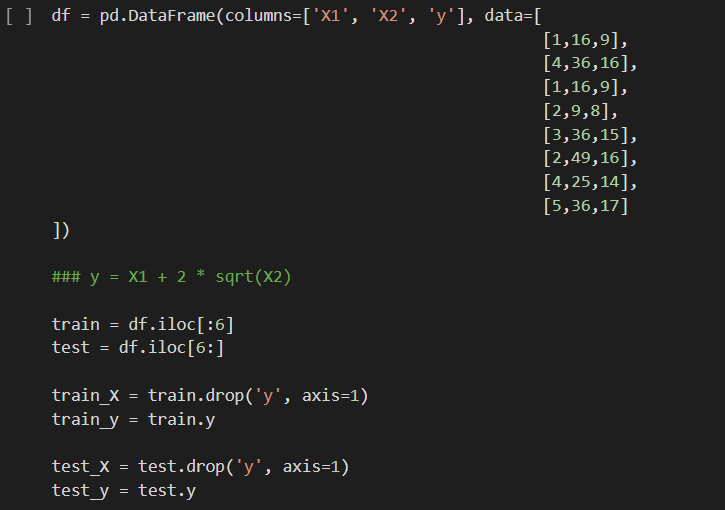Create DataFrame

To understand the examples better, we’ll create a dataset that will help us explore the code better.

The code above creates data which follows the equation `y = X1 + 2 * sqrt(X2)`. This makes sure a simple Linear Regression model is not able to fit it perfectly.

Let’s see what prediction results are thrown at us: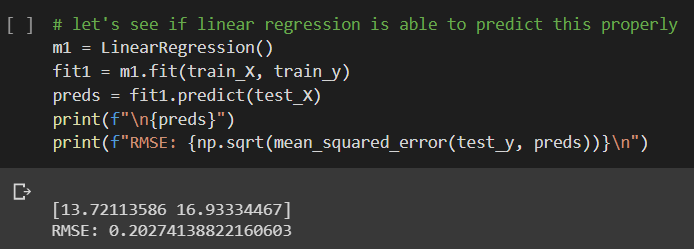LinearRegression predictions on raw data

A perfect prediction would be 14 and 17. The predictions are not bad, but can we do some calculations on the input features to make this better?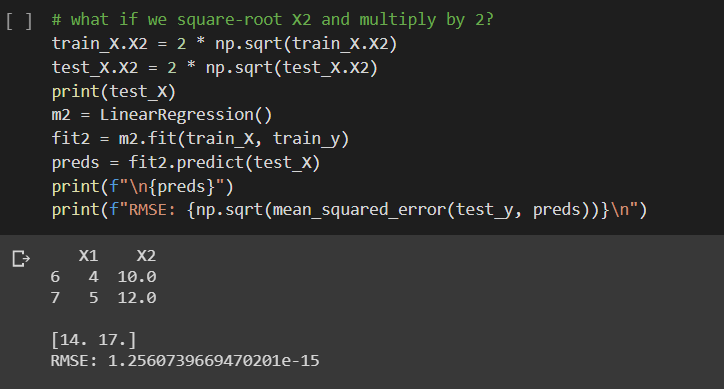Predictions after input feature manipulation

The input manipulations cause it to fit a perfect linear trend (`y=X1+X2` now), and hence the perfect predictions. Now, this is just an example, but suppose for a dataset, your analysis said such input transformation would be good, how do you do that in a safe manner via Pipelines.

Let’s see a basic LinearRegression() model fitted by using a Pipeline.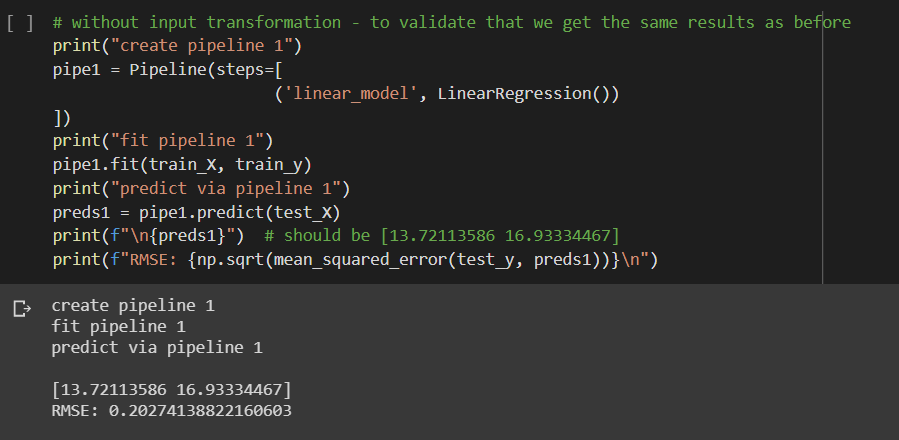LinearRegression() with Pipeline

1. We declare a `pipe1` variable using `Pipeline` class with array of `steps` inside it. The name of the step (in this case `linear_model`) could be anything unique of your choice. It is followed by an actual Transformer or Estimator (in this case, our `LinearRegression()` model).
2. Like any other model, it is fitted on the training data, but using the `pipe1` variable.
3. Use `pipe1` to predict on test set as you would do in any other model.

To perform the input calculations/transformations, we’ll design a custom transformer.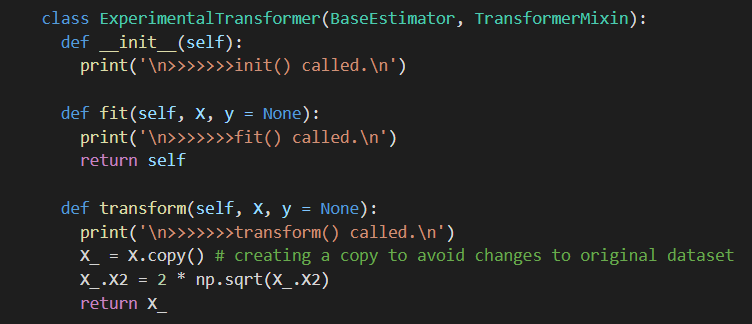Custom Input Transformer

We create a class and name it `ExperimentalTransformer`. All transformers we design will inherit from `BaseEstimator` and `TransformerMixin` classes as they give us pre-existing methods for free. You can read more about them in the article links I provided above.

There are 3 methods to take care of here:

1. `__init__` : This is the constructor. Called when pipeline is initialized.
2. `fit()` : Called when we fit the pipeline.
3. `transform()` : Called when we use fit or transform on the pipeline.

For the moment, let’s just put print() messages in init & fit(), and write our calculations in transform(). As you see above, we return the modified values there. All the input features will be passed into `X` when fit() or transform() is called.

Let’s put this into a pipeline to see the order in which these functions are called.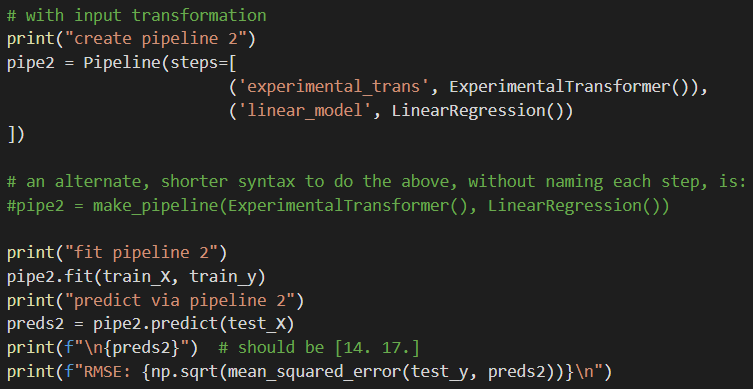ExperimentalTransformer in Pipeline

You can see in the code comments above, one can also use make_pipeline() syntax, which is shorter, to create pipelines.

Now the output: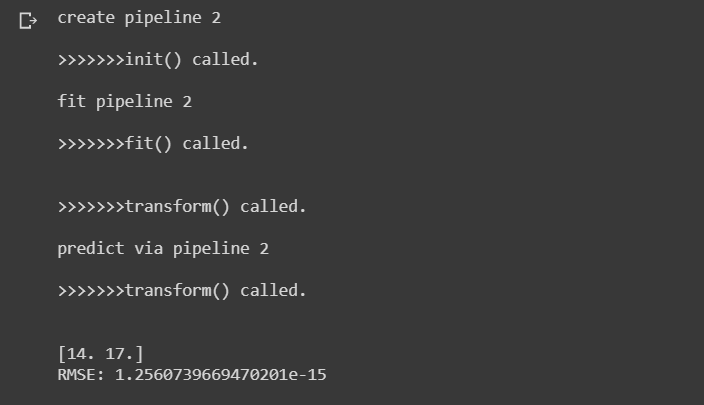Output with ExperimentalTransformer

3 important things to note:

a. init was called the moment we initialized the `pipe2` variable.

b. Both fit() and transform() of our ExperimentalTransformer were called when we fitted the pipeline on training data. This makes sense as that is how model fitting works. You would need to transform input features while trying to predict train_y.

c. transform() is called, as expected, when we call predict(test_X) — the input test features need to be square-rooted and doubled too before making predictions.

The result — perfect predictions!

## Hire Machine Learning Developers in India

We supply you with world class machine learning experts / ML Developers with years of domain experience who can add more value to your business.

## The Data Science & Machine Learning Bootcamp in Python

Learn Data Science, Machine Learning, and Deep Learning. In this article, I’ll show you how you stand to benefit by taking my data science course.

## Data Science Projects | Data Science | Machine Learning | Python

Practice your skills in Data Science with Python, by learning and then trying all these hands-on, interactive projects, that I have posted for you.

## Data Science Projects | Data Science | Machine Learning | Python

Practice your skills in Data Science with Python, by learning and then trying all these hands-on, interactive projects, that I have posted for you.

## Data Science Projects | Data Science | Machine Learning | Python

Practice your skills in Data Science with Python, by learning and then trying all these hands-on, interactive projects, that I have posted for you.